PSABM4Z3 - Practice---Acc7.3 Lesson 13 Using Equations to Solve for Unknown Angles (7.EE.B.4, 7.G.B.5

Segments ABDC, and EC intersect at point C. Angle DCE measures 148. Find the value of x.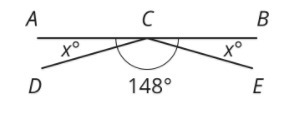x = _____

Type your answer below as a number (example: 5, 3.1, 4 1/2, or 3/2):
Part A)

Line  is perpendicular to line m. Find the value of x and w.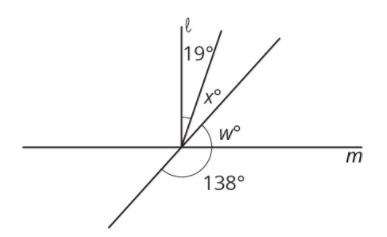w = _____

Type your answer below as a number (example: 5, 3.1, 4 1/2, or 3/2):
Part B)

x = _____

Type your answer below as a number (example: 5, 3.1, 4 1/2, or 3/2):
Part A)

If you knew that two angles were complementary and were given the measure of one of those angles, would you be able to find the measure of the other angle?

Select one:
Part B)

Part A)

Here is a polygon on a grid.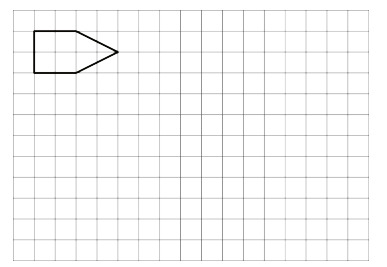Draw a scaled copy of the polygon using a scale factor 3. Label the copy A.

Draw a scaled copy of the polygon with a scale factor. Label it B.

Draw both images on paper, take a picture, and upload it using the "insert image" icon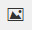.  If you do not have the ability to upload an image of your work, type "Images on paper."

Part B)

Is polygon A a scaled copy of Polygon B?

Select one:
Part C)

What is the scale factor that takes B to A?

Type your answer below as a number (example: 5, 3.1, 4 1/2, or 3/2):
Part A)

Jada, Elena, and Lin walked a total of 37 miles last week. Jada walked 4 more miles than Elena, and Lin walked 2 more miles than Jada. The diagram represents this situation: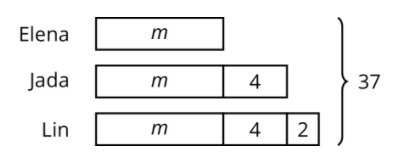Find the number of miles that they each walked.

How many miles did Elena walk?

Type your answer below as a number (example: 5, 3.1, 4 1/2, or 3/2):
Part B)

How many miles did Jada walk?

Type your answer below as a number (example: 5, 3.1, 4 1/2, or 3/2):
Part C)

How many miles did Lin walk?

Type your answer below as a number (example: 5, 3.1, 4 1/2, or 3/2):
Part D)